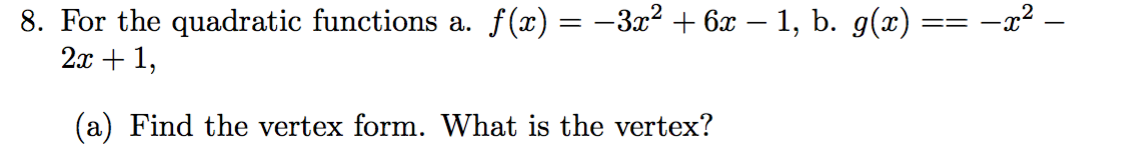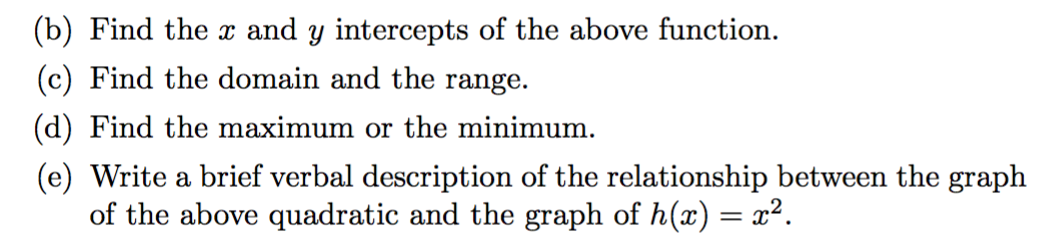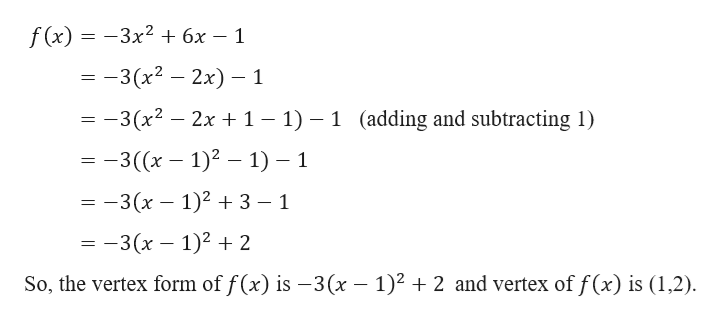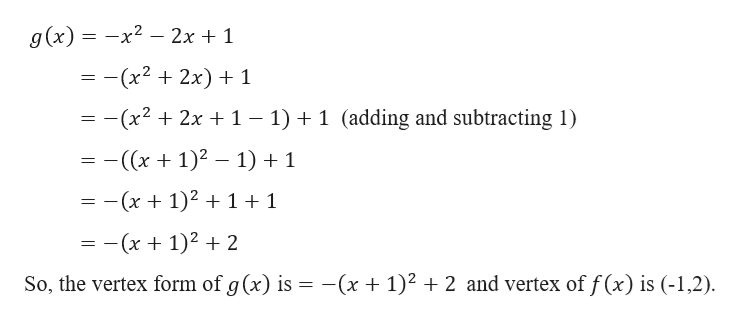# 8. For the quadratic functions a. f(x) = -3z2 6x - 1, b. g(x) =2x1(a) Find the vertex form. What is the vertex? (b) Find the x and y intercepts of the above function.(c) Find the domain and the range(d) Find the maximum or the minimum.(e) Write a brief verbal description of the relationship between the graphof the above quadratic and the graph of h(x) x2.

Question
2 viewshelp_outlineImage Transcriptionclose8. For the quadratic functions a. f(x) = -3z2 6x - 1, b. g(x) = 2x1 (a) Find the vertex form. What is the vertex? fullscreenhelp_outlineImage Transcriptionclose(b) Find the x and y intercepts of the above function. (c) Find the domain and the range (d) Find the maximum or the minimum. (e) Write a brief verbal description of the relationship between the graph of the above quadratic and the graph of h(x) x2. fullscreen
check_circle

Step 1

As per our policy I am solving 1st three subparts and for the remaining subparts post the question again separately.

Given,

Step 2

Part ahelp_outlineImage Transcriptionclose3x2 + 6x - 1 f (x) -3(x2 — 2х) — 1 3(x2 2x 1 - 1) 1 (adding and subtracting 1) - -3((x -1)2 -1) -1 — — 3 (х — 1)2 + 3 — 1 — — 3 (х — 1)2 + 2 So, the vertex form of f(x) is -3(x -1)2 2 and vertex of f(x) is (1,2) fullscreen
Step 3

And

...help_outlineImage Transcriptioncloseg(x)-x2 - 2x + 1 - -(x2 2x) 1 -(x2 2x 1- 1)+ 1 (adding and subtracting 1) ((1)2 1) + 1 -(x + 1)2 + 1 +1 = -(x + 1)2 + 2 -(x + 1)2 2 and vertex of f (x) is (-1,2) So, the vertex form of g (x) is fullscreen

### Want to see the full answer?

See Solution

#### Want to see this answer and more?

Solutions are written by subject experts who are available 24/7. Questions are typically answered within 1 hour.*

See Solution
*Response times may vary by subject and question.
Tagged in

### Functions